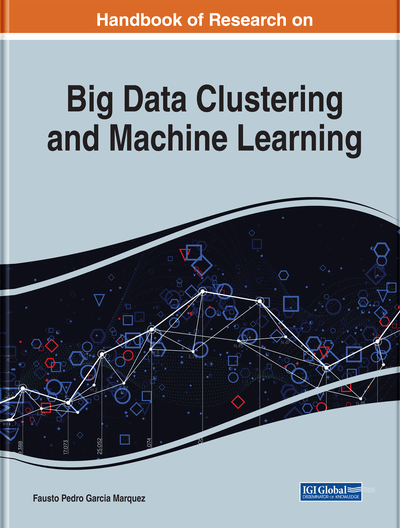# Modern Statistical Modeling in Machine Learning and Big Data Analytics: Statistical Models for Continuous and Categorical Variables

Niloofar Ramezani (George Mason University, USA)
DOI: 10.4018/978-1-7998-0106-1.ch007

## Abstract

Machine learning, big data, and high dimensional data are the topics we hear about frequently these days, and some even call them the wave of the future. Therefore, it is important to use appropriate statistical models, which have been established for many years, and their efficiency has already been evaluated to contribute into advancing machine learning, which is a relatively newer field of study. Different algorithms that can be used within machine learning, depending on the nature of the variables, are discussed, and appropriate statistical techniques for modeling them are presented in this chapter.
Chapter Preview
Top

## Continuous Data

When dealing with continuous data and predicting such outcome measures, regression approaches and algorithms can be used. Linear regression is by far the simplest and most popular example of a regression algorithm used in many fields. Though it is often underrated due to its simplicity, it is a flexible prediction tool. Regression trees and support vector regressions are more advanced algorithms within the framework of regression that can be used for high dimensions of data.

## Complete Chapter List

Search this Book:
Reset